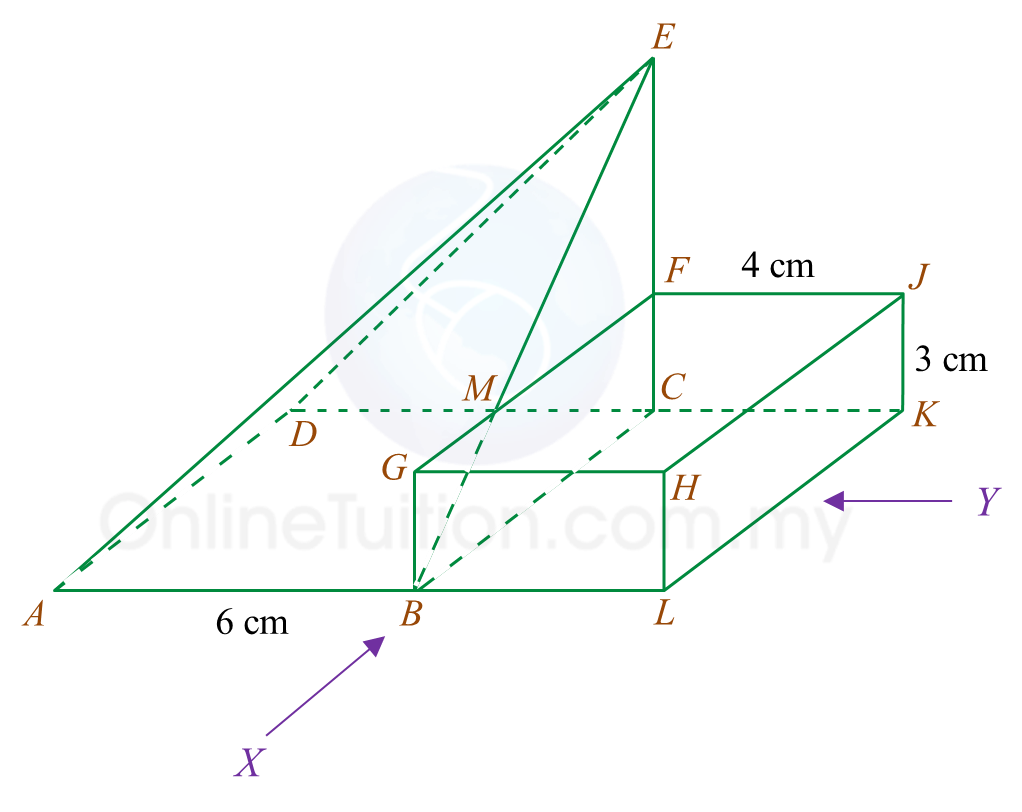# SPM Mathematics 2016, Paper 2 (Questions 15 & 16)

Question 15 (12 marks):
You are not allowed to use graph paper to answer this question.

(a)
Diagram 15.1 shows a pyramid with rectangular base ABCD on a horizontal plane. Vertex E is vertically above C. Triangles BCE and DCE are vertical planes. Triangles ABE and ADE are inclined planes.Diagram 15.1

Draw to full scale, the plan of the solid.

(b)
Another solid cuboid with rectangle base BLKC is joined to the pyramid in Diagram 15.1 at the vertical plane BCFM. The composite solid is as shown in Diagram 15.2. The base ABLKCD lies on a horizontal planeDiagram 15.2

Draw to full scale,
(i) the elevation of the composite solid on a vertical plane parallel to ABL as viewed from X.

(ii)
the elevation of the composite solid on a vertical plane parallel to LK as viewed from Y.

Solution:

(a)(b)(i)(b)(ii)Question 16 (12 marks):
A(25o N, 35o E), B(25o N, 40o W), C and D are four points which lie on the surface of the earth. AD is the diameter of the common parallel latitude 25o N .
(a) Find the longitude of D.

(b)
C lies 3300 nautical miles due south of measured along the surface of the earth.
Calculate the latitude of C.

(c)
Calculate the shortest distance, in nautical mile, from to measured along the surface of the earth.

(d)
An aeroplane took off from C and flew due north to point A.
The total time taken for the whole flight was 12 hours 24 minutes.

(i)
Calculate the distance, in nautical mile, from A due west to measured along the common parallel of latitude.

(ii)
Calculate the average speed, in knot, of the whole flight.

Solution:
(a)
Longitude of D = (180o – 35o)W
= 145oW

(b)

(c)
Shortest distance of to D
= (65o + 65o) × 60’
= 130o × 60’
= 7800 nautical miles

(d)(i)
Distance from A to B
= (35o + 40o) × 60’ × cos 25o
= 75o × 60’ × cos 25o
= 4078.4 nautical miles

(d)(ii)